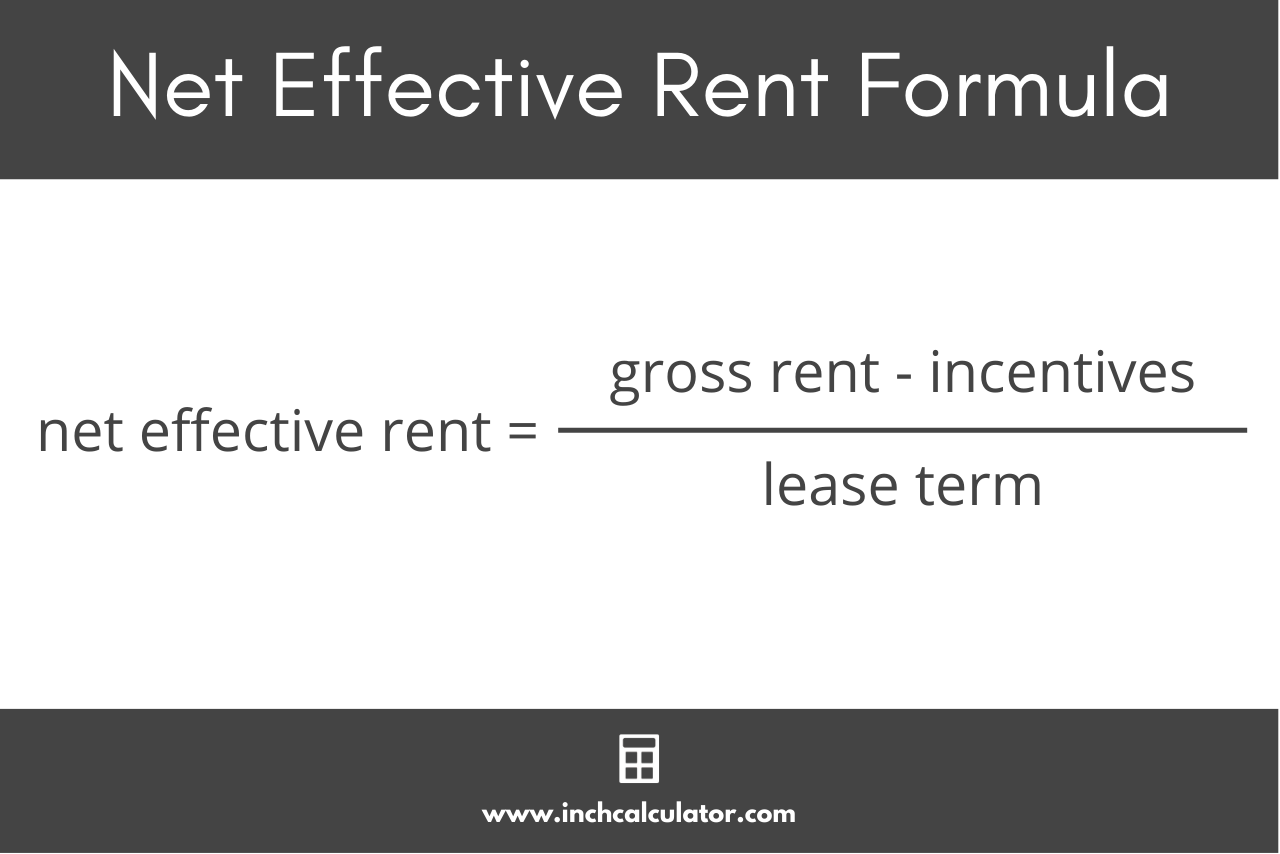# Net Effective Rent Calculator

Calculate the net effective rent for an apartment or lease after incentives, free months, or fixed discounts using the calculator below.

\$
Concessions and Discounts
\$

## Net Effective Rent:

 \$ per Month Annual
Learn how we calculated this below

## How to Calculate Net Effective Rent

When managing a property or renting an apartment, it’s important to understand the concept of net effective rent. Net effective rent is the average amount a tenant pays in rent each month after factoring in any incentives or discounts provided by the landlord.

Incentives can include things such as free months of rent, waived fees, or improvements to the space. Calculating net effective rent is necessary to understand the actual rent being paid after granting incentives.

Net effective rent is different from prorated rent in that instead of being a partial month’s rent, it’s actually the average rent paid per month.

### Net Effective Rent Formula

You can calculate the net effective rent using the following formula:

net effective rent = gross rent – incentives / lease term

Thus, the net effective rent per month is equal to the gross rent for the lease term minus any incentives, divided by the lease term.You can also use the net effective rent when calculating the annual rent income for a rental property.

For example, let’s calculate the net effective rent for an apartment with a monthly rent of \$1,500 for a 12-month lease term after a one-free-month incentive for signing the lease.

net effective rent = (\$1,500 × 12) – \$1,500 / 12
net effective rent = \$18,000 – \$1,500 / 12
net effective rent = \$16,500 / 12
net effective rent = \$1,375

So, in this example, the net effective rent is \$1,375 per month.